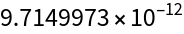Function Repository Resource:

Get a numerically sorted list of abscissa-weight pairs for generalized Gaussian quadrature

Contributed by: Jan Mangaldan
 ResourceFunction["GeneralizedGaussianQuadratureWeights"][n,poly] gives a list of the n pairs {xi,wi} of the elementary n-point Gaussian quadrature formula associated with the orthogonal polynomial represented by poly, where wi is the weight of the abscissa xi. ResourceFunction["GeneralizedGaussianQuadratureWeights"][n,poly,prec] uses the working precision prec.

## Details

Gaussian quadrature approximates the value of an integral as a linear combination of values of the integrand evaluated at optimal abscissas xi: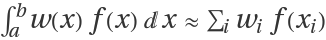, where w(x) and (a,b) are the weight function and orthogonality interval associated with the specified orthogonal polynomial.
The weights in Gaussian quadrature are sometimes referred to as Christoffel numbers or Cotes numbers.
The argument poly can be any of the following, with the corresponding integral type being approximated:
 "ChebyshevFirst" Gauss–Chebyshev quadrature of the first kind,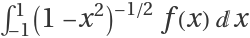"ChebyshevSecond" Gauss–Chebyshev quadrature of the second kind,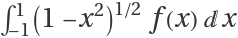"Hermite" Gauss–Hermite quadrature,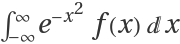"Laguerre" Gauss–Laguerre quadrature,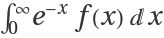"Legendre" Gauss–Legendre quadrature,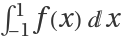{"Gegenbauer",m} Gauss–Gegenbauer quadrature,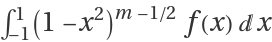{"Laguerre",a} generalized Gauss–Laguerre quadrature,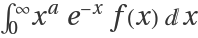{"Jacobi",a,b} Gauss–Jacobi quadrature,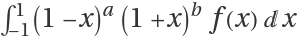Strings in specifications like "Legendre" and {"Jacobi",a,b} can be replaced with the corresponding built-in symbols, as in LegendreP and {JacobiP,a,b}.
The precision argument acts similarly to the WorkingPrecision option used in many Wolfram Language numeric functions; it is not at all like the PrecisionGoal option. If the n argument is too small to allow for a high-precision result, extra precision in a result will not be meaningful.

## Examples

### Basic Examples (2)

Generate the abscissas and weights for three-point Gauss–Legendre quadrature:

 In:=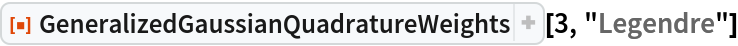Out=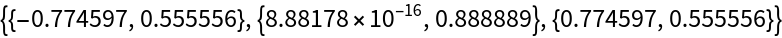Use 25-digit precision:

 In:=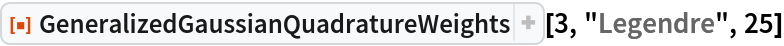Out=### Scope (2)

Generate a four-point Gauss-Jacobi quadrature with specific values of a and b:

 In:=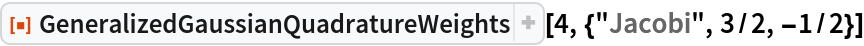Out=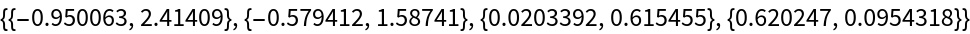An equivalent specification:

 In:=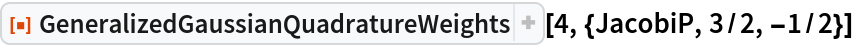Out=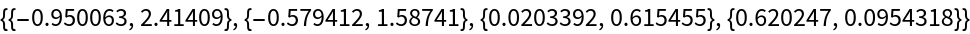### Applications (2)

Use generalized Gauss–Laguerre quadrature to approximate the integral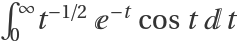:

 In:=Out=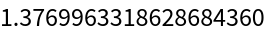In:=Out=